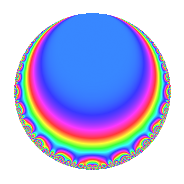# Properties

 Label 4003.2.a.aLevel 4003 Weight 2 Character orbit 4003.a Self dual Yes Analytic conductor 31.964 Analytic rank 1 Dimension 2 CM No Inner twists 1

# Related objects

## Newspace parameters

 Level: $$N$$ = $$4003$$ Weight: $$k$$ = $$2$$ Character orbit: $$[\chi]$$ = 4003.a (trivial)

## Newform invariants

 Self dual: Yes Analytic conductor: $$31.9641159291$$ Analytic rank: $$1$$ Dimension: $$2$$ Coefficient field: $$\Q(\sqrt{2})$$ Coefficient ring: $$\Z[a_1, a_2]$$ Coefficient ring index: $$1$$ Fricke sign: $$1$$ Sato-Tate group: $\mathrm{SU}(2)$

## $q$-expansion

Coefficients of the $$q$$-expansion are expressed in terms of $$\beta = \sqrt{2}$$. We also show the integral $$q$$-expansion of the trace form.

 $$f(q)$$ $$=$$ $$q$$ $$+ \beta q^{2}$$ $$+ \beta q^{3}$$ $$+ \beta q^{5}$$ $$+ 2 q^{6}$$ $$- q^{7}$$ $$-2 \beta q^{8}$$ $$- q^{9}$$ $$+O(q^{10})$$ $$q$$ $$+ \beta q^{2}$$ $$+ \beta q^{3}$$ $$+ \beta q^{5}$$ $$+ 2 q^{6}$$ $$- q^{7}$$ $$-2 \beta q^{8}$$ $$- q^{9}$$ $$+ 2 q^{10}$$ $$+ ( -2 - 2 \beta ) q^{11}$$ $$+ ( 4 + 2 \beta ) q^{13}$$ $$-\beta q^{14}$$ $$+ 2 q^{15}$$ $$-4 q^{16}$$ $$+ ( -1 - 4 \beta ) q^{17}$$ $$-\beta q^{18}$$ $$+ ( 1 + 4 \beta ) q^{19}$$ $$-\beta q^{21}$$ $$+ ( -4 - 2 \beta ) q^{22}$$ $$+ ( -6 + 2 \beta ) q^{23}$$ $$-4 q^{24}$$ $$-3 q^{25}$$ $$+ ( 4 + 4 \beta ) q^{26}$$ $$-4 \beta q^{27}$$ $$+ ( -4 - 2 \beta ) q^{29}$$ $$+ 2 \beta q^{30}$$ $$+ ( 2 - 6 \beta ) q^{31}$$ $$+ ( -4 - 2 \beta ) q^{33}$$ $$+ ( -8 - \beta ) q^{34}$$ $$-\beta q^{35}$$ $$+ ( -7 - 2 \beta ) q^{37}$$ $$+ ( 8 + \beta ) q^{38}$$ $$+ ( 4 + 4 \beta ) q^{39}$$ $$-4 q^{40}$$ $$+ ( 8 + 2 \beta ) q^{41}$$ $$-2 q^{42}$$ $$-\beta q^{45}$$ $$+ ( 4 - 6 \beta ) q^{46}$$ $$+ ( 4 + 5 \beta ) q^{47}$$ $$-4 \beta q^{48}$$ $$-6 q^{49}$$ $$-3 \beta q^{50}$$ $$+ ( -8 - \beta ) q^{51}$$ $$+ ( -2 - 8 \beta ) q^{53}$$ $$-8 q^{54}$$ $$+ ( -4 - 2 \beta ) q^{55}$$ $$+ 2 \beta q^{56}$$ $$+ ( 8 + \beta ) q^{57}$$ $$+ ( -4 - 4 \beta ) q^{58}$$ $$+ ( -6 + 2 \beta ) q^{59}$$ $$+ ( 10 + 2 \beta ) q^{61}$$ $$+ ( -12 + 2 \beta ) q^{62}$$ $$+ q^{63}$$ $$+ 8 q^{64}$$ $$+ ( 4 + 4 \beta ) q^{65}$$ $$+ ( -4 - 4 \beta ) q^{66}$$ $$+ ( 7 - 4 \beta ) q^{67}$$ $$+ ( 4 - 6 \beta ) q^{69}$$ $$-2 q^{70}$$ $$+ 6 q^{71}$$ $$+ 2 \beta q^{72}$$ $$-3 q^{73}$$ $$+ ( -4 - 7 \beta ) q^{74}$$ $$-3 \beta q^{75}$$ $$+ ( 2 + 2 \beta ) q^{77}$$ $$+ ( 8 + 4 \beta ) q^{78}$$ $$+ ( 3 - 6 \beta ) q^{79}$$ $$-4 \beta q^{80}$$ $$-5 q^{81}$$ $$+ ( 4 + 8 \beta ) q^{82}$$ $$+ ( 3 - 10 \beta ) q^{83}$$ $$+ ( -8 - \beta ) q^{85}$$ $$+ ( -4 - 4 \beta ) q^{87}$$ $$+ ( 8 + 4 \beta ) q^{88}$$ $$+ ( -9 + 2 \beta ) q^{89}$$ $$-2 q^{90}$$ $$+ ( -4 - 2 \beta ) q^{91}$$ $$+ ( -12 + 2 \beta ) q^{93}$$ $$+ ( 10 + 4 \beta ) q^{94}$$ $$+ ( 8 + \beta ) q^{95}$$ $$+ ( 6 + 3 \beta ) q^{97}$$ $$-6 \beta q^{98}$$ $$+ ( 2 + 2 \beta ) q^{99}$$ $$+O(q^{100})$$ $$\operatorname{Tr}(f)(q)$$ $$=$$ $$2q$$ $$\mathstrut +\mathstrut 4q^{6}$$ $$\mathstrut -\mathstrut 2q^{7}$$ $$\mathstrut -\mathstrut 2q^{9}$$ $$\mathstrut +\mathstrut O(q^{10})$$ $$2q$$ $$\mathstrut +\mathstrut 4q^{6}$$ $$\mathstrut -\mathstrut 2q^{7}$$ $$\mathstrut -\mathstrut 2q^{9}$$ $$\mathstrut +\mathstrut 4q^{10}$$ $$\mathstrut -\mathstrut 4q^{11}$$ $$\mathstrut +\mathstrut 8q^{13}$$ $$\mathstrut +\mathstrut 4q^{15}$$ $$\mathstrut -\mathstrut 8q^{16}$$ $$\mathstrut -\mathstrut 2q^{17}$$ $$\mathstrut +\mathstrut 2q^{19}$$ $$\mathstrut -\mathstrut 8q^{22}$$ $$\mathstrut -\mathstrut 12q^{23}$$ $$\mathstrut -\mathstrut 8q^{24}$$ $$\mathstrut -\mathstrut 6q^{25}$$ $$\mathstrut +\mathstrut 8q^{26}$$ $$\mathstrut -\mathstrut 8q^{29}$$ $$\mathstrut +\mathstrut 4q^{31}$$ $$\mathstrut -\mathstrut 8q^{33}$$ $$\mathstrut -\mathstrut 16q^{34}$$ $$\mathstrut -\mathstrut 14q^{37}$$ $$\mathstrut +\mathstrut 16q^{38}$$ $$\mathstrut +\mathstrut 8q^{39}$$ $$\mathstrut -\mathstrut 8q^{40}$$ $$\mathstrut +\mathstrut 16q^{41}$$ $$\mathstrut -\mathstrut 4q^{42}$$ $$\mathstrut +\mathstrut 8q^{46}$$ $$\mathstrut +\mathstrut 8q^{47}$$ $$\mathstrut -\mathstrut 12q^{49}$$ $$\mathstrut -\mathstrut 16q^{51}$$ $$\mathstrut -\mathstrut 4q^{53}$$ $$\mathstrut -\mathstrut 16q^{54}$$ $$\mathstrut -\mathstrut 8q^{55}$$ $$\mathstrut +\mathstrut 16q^{57}$$ $$\mathstrut -\mathstrut 8q^{58}$$ $$\mathstrut -\mathstrut 12q^{59}$$ $$\mathstrut +\mathstrut 20q^{61}$$ $$\mathstrut -\mathstrut 24q^{62}$$ $$\mathstrut +\mathstrut 2q^{63}$$ $$\mathstrut +\mathstrut 16q^{64}$$ $$\mathstrut +\mathstrut 8q^{65}$$ $$\mathstrut -\mathstrut 8q^{66}$$ $$\mathstrut +\mathstrut 14q^{67}$$ $$\mathstrut +\mathstrut 8q^{69}$$ $$\mathstrut -\mathstrut 4q^{70}$$ $$\mathstrut +\mathstrut 12q^{71}$$ $$\mathstrut -\mathstrut 6q^{73}$$ $$\mathstrut -\mathstrut 8q^{74}$$ $$\mathstrut +\mathstrut 4q^{77}$$ $$\mathstrut +\mathstrut 16q^{78}$$ $$\mathstrut +\mathstrut 6q^{79}$$ $$\mathstrut -\mathstrut 10q^{81}$$ $$\mathstrut +\mathstrut 8q^{82}$$ $$\mathstrut +\mathstrut 6q^{83}$$ $$\mathstrut -\mathstrut 16q^{85}$$ $$\mathstrut -\mathstrut 8q^{87}$$ $$\mathstrut +\mathstrut 16q^{88}$$ $$\mathstrut -\mathstrut 18q^{89}$$ $$\mathstrut -\mathstrut 4q^{90}$$ $$\mathstrut -\mathstrut 8q^{91}$$ $$\mathstrut -\mathstrut 24q^{93}$$ $$\mathstrut +\mathstrut 20q^{94}$$ $$\mathstrut +\mathstrut 16q^{95}$$ $$\mathstrut +\mathstrut 12q^{97}$$ $$\mathstrut +\mathstrut 4q^{99}$$ $$\mathstrut +\mathstrut O(q^{100})$$

## Embeddings

For each embedding $$\iota_m$$ of the coefficient field, the values $$\iota_m(a_n)$$ are shown below.

For more information on an embedded modular form you can click on its label.

Label $$\iota_m(\nu)$$ $$a_{2}$$ $$a_{3}$$ $$a_{4}$$ $$a_{5}$$ $$a_{6}$$ $$a_{7}$$ $$a_{8}$$ $$a_{9}$$ $$a_{10}$$
1.1
 −1.41421 1.41421
−1.41421 −1.41421 0 −1.41421 2.00000 −1.00000 2.82843 −1.00000 2.00000
1.2 1.41421 1.41421 0 1.41421 2.00000 −1.00000 −2.82843 −1.00000 2.00000
 $$n$$: e.g. 2-40 or 990-1000 Significant digits: Format: Complex embeddings Normalized embeddings Satake parameters Satake angles

## Inner twists

This newform does not admit any (nontrivial) inner twists.

## Atkin-Lehner signs

$$p$$ Sign
$$4003$$ $$1$$

## Hecke kernels

This newform can be constructed as the kernel of the linear operator $$T_{2}^{2}$$ $$\mathstrut -\mathstrut 2$$ acting on $$S_{2}^{\mathrm{new}}(\Gamma_0(4003))$$.